# Collineation

projective

A projective transformation (projective isomorphism) of a projective space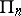that is representable as the product of a finite number of perspectivities (cf. Perspective); ifis a projective collineation, then for any subspace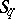there exists a productof not more than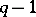perspectivities such thatfor any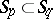. For example, a projective transformation that leaves each point of some straight line fixed is a collineation, this is, a homology (in the narrow sense).

Letbe interpreted as the collection of subspaces of the linear spaceover a skew-field. Then in order that a projective transformation be a projective collineation, it is necessary and sufficient that it be induced by a linear transformation of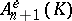. The collection of all projective collineations forms a subgroup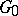of the group of projective transformationswhich is a normal subgroup of.

The projective collineations exhaust all the projective transformations if and only if every automorphism of the skew-fieldis inner. A field possesses this property if and only if any of its automorphisms is the identity, such as, for example, the field of real numbers. The complex fielddoes not possess this property, whereas every automorphism of the skew-field of quaternions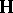is inner.

Ifis a non-commutative skew-field, then there exists a non-trivial projective collineation that leaves every point of a given simplex fixed. Each simplex can be mapped onto any other simplex by precisely one projective collineation, if and only ifis a field (the second fundamental theorem of projective geometry).

There is a large amount of confusion in the literature on projective geometry about the terminology for the different kinds of transformations. The transformation defined above, a projective collineation, sometimes also called a projectivity, is usually defined as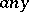transformation that is the product of a finite number of perspectivities. Some authors use the term projectivity, however, only for mappings between lines that are the product of a finite number of perspectivities. A projective transformation, usually called a projective isomorphism, or in older literature simply a collineation, is a bijection between projective spaces that preserves incidence.